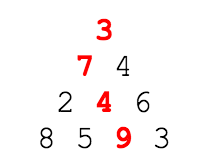# Project Euler

### A Taste of Number Theory

#### Problem 67: Maximum Path Sum 2

By starting at the top of the triangle below and moving to adjacent numbers on the row below, the maximum total from top to bottom is 23.That is, 3 + 7 + 4 + 9 = 23.

Find the maximum total from top to bottom in triangle.txt (right click and 'Save Link/Target As...'), a 15K text file containing a triangle with one-hundred rows.

NOTE: This is a much more difficult version of Problem 18. It is not possible to try every route to solve this problem, as there are 299 altogether! If you could check one trillion (1012) routes every second it would take over twenty billion years to check them all. There is an efficient algorithm to solve it.)

#### The Light

Check Problem 18 discussion on how to solve this type of problem.

#### The Code

```import java.io.*;
import java.util.*;

public class Problem67
{
public static void main(String[] args) throws FileNotFoundException
{
int row = 100;
int rowIndex = 0;
int[][] tri = new int[row][];

Scanner scan = new Scanner(new File("triangle.txt"));
while(scan.hasNextLine())
{
String s = scan.nextLine();
String[] input = s.split(" ");

tri[rowIndex] = new int[input.length];
for(int i = 0; i < input.length; i++)
{
tri[rowIndex][i] = Integer.parseInt(input[i]);
}
rowIndex++;
}
for(int i = row - 1; i > 0; i--)
{
int index = 0;
for(int j = 0; j < tri[i].length - 1; j++, index++)
{
int tmp1 = tri[i][j] + tri[i - 1][index];
int tmp2 = tri[i][j + 1] + tri[i - 1][index];
if(tmp1 > tmp2)
tri[i - 1][index] = tmp1;
else
tri[i - 1][index] = tmp2;
}
}
System.out.println("Maximum total from top to bottom: " + tri);
}
}
```